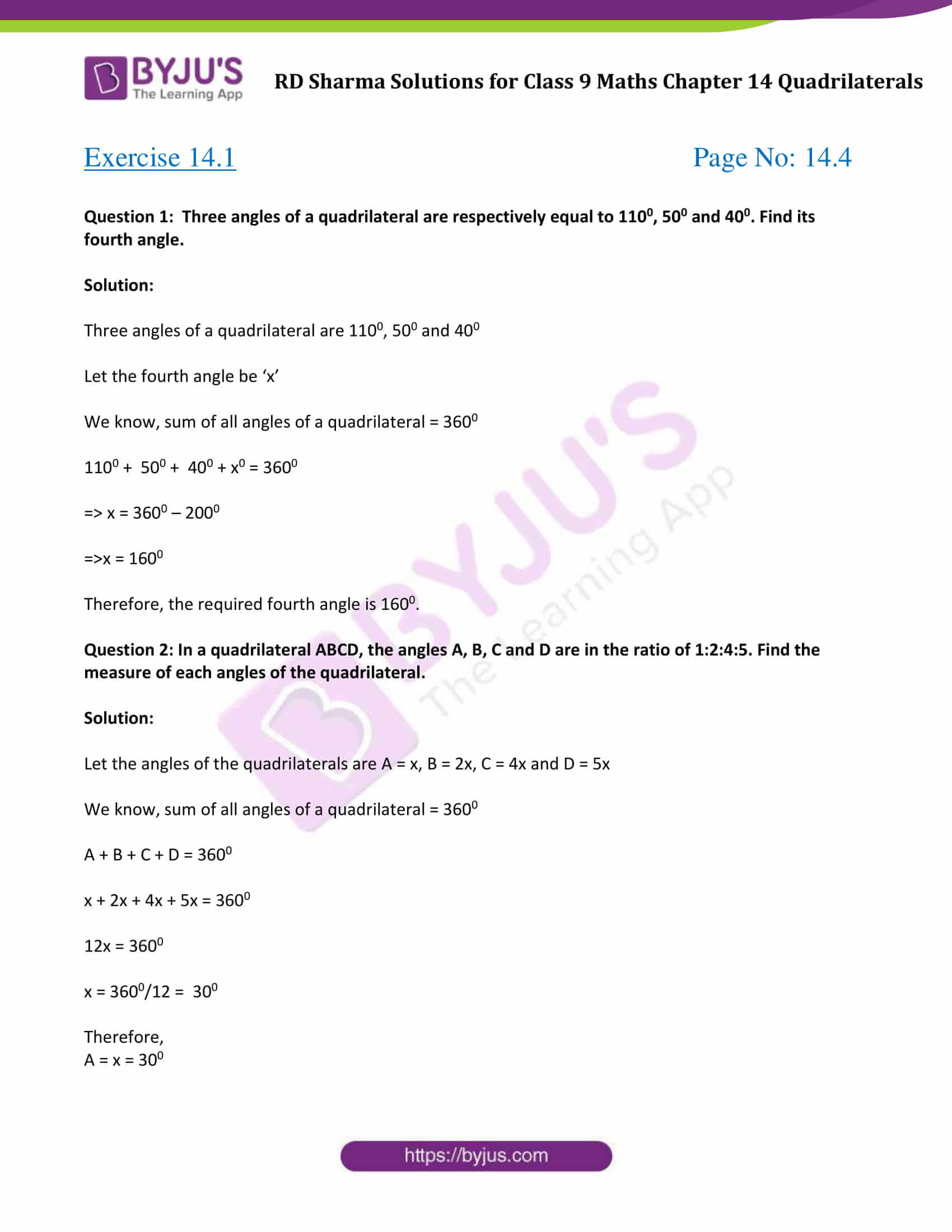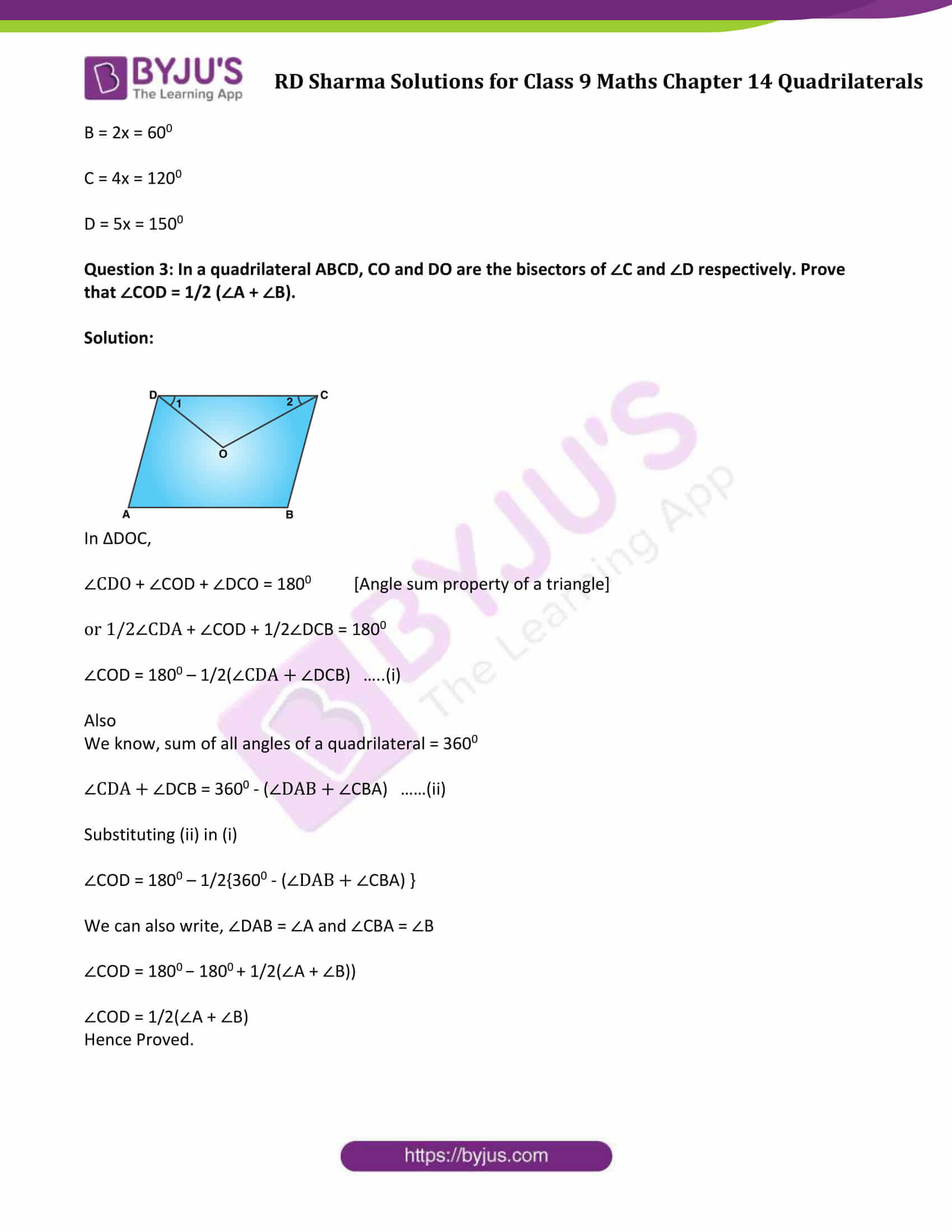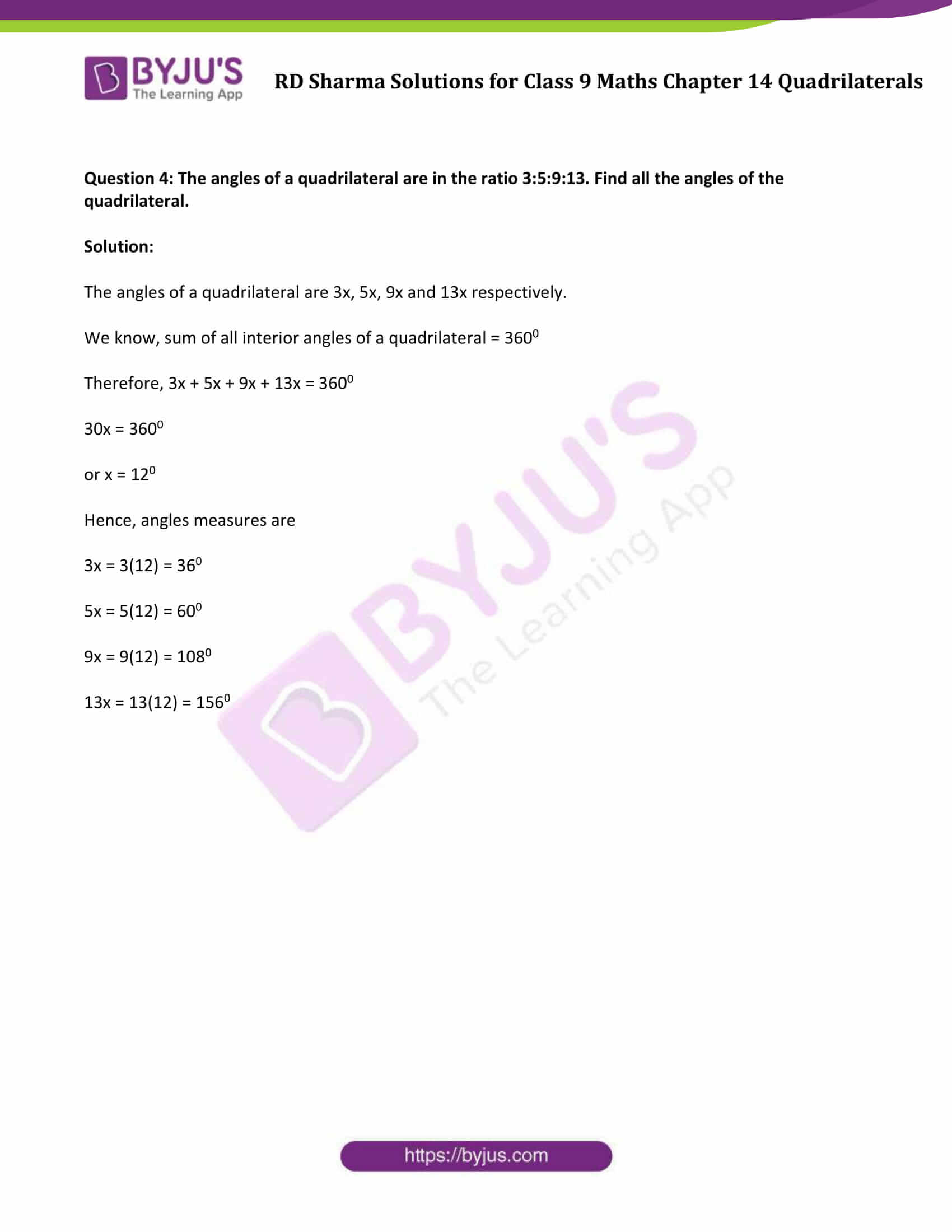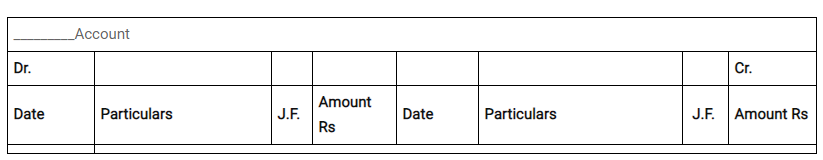# RD Sharma Solutions for Class 9 Maths Chapter 14 Quadrilaterals Exercise 14.1

RD Sharma Class 9 Mathematics Chapter 14 Exercise 14.1 Solutions for Quadrilaterals are provided here. In this exercise, students will learn about quadrilaterals angles, angle sum property of a quadrilateral and many more. RD Sharma Solutions class 9 offers an effortful challenge to students trying to get their concepts straightened out. With a strong foundation in all major Mathematical topics will help you be ready to solve the hardest of algebraic problems.### Access Answers to Maths RD Sharma Solutions for Class 9 Chapter 14 Quadrilaterals Exercise 14.1 Page number 14.4

Question 1: Three angles of a quadrilateral are respectively equal to 1100, 500 and 400. Find its fourth angle.

Solution:

Three angles of a quadrilateral are 1100, 500 and 400

Let the fourth angle be ‘x’

We know, sum of all angles of a quadrilateral = 3600

1100 + 500 + 400 + x0 = 3600

⇒ x = 3600 – 2000

⇒x = 1600

Therefore, the required fourth angle is 1600.

Question 2: In a quadrilateral ABCD, the angles A, B, C and D are in the ratio of 1:2:4:5. Find the measure of each angles of the quadrilateral.

Solution:

Let the angles of the quadrilaterals are A = x, B = 2x, C = 4x and D = 5x

We know, sum of all angles of a quadrilateral = 3600

A + B + C + D = 3600

x + 2x + 4x + 5x = 3600

12x = 3600

x = 3600/12 = 300

Therefore,

A = x = 300

B = 2x = 600

C = 4x = 1200

D = 5x = 1500

Question 3: In a quadrilateral ABCD, CO and DO are the bisectors of ∠C and ∠D respectively. Prove that ∠COD = 1/2 (∠A + ∠B).

Solution:In ΔDOC,

∠CDO + ∠COD + ∠DCO = 1800 [Angle sum property of a triangle]

or 1/2∠CDA + ∠COD + 1/2∠DCB = 1800

∠COD = 1800 – 1/2(∠CDA + ∠DCB) …..(i)

Also

We know, sum of all angles of a quadrilateral = 3600

∠CDA + ∠DCB = 3600 – (∠DAB + ∠CBA) ……(ii)

Substituting (ii) in (i)

∠COD = 1800 – 1/2{3600 – (∠DAB + ∠CBA) }

We can also write, ∠DAB = ∠A and ∠CBA = ∠B

∠COD = 1800 − 1800 + 1/2(∠A + ∠B))

∠COD = 1/2(∠A + ∠B)

Hence Proved.

Question 4: The angles of a quadrilateral are in the ratio 3:5:9:13. Find all the angles of the quadrilateral.

Solution:

The angles of a quadrilateral are 3x, 5x, 9x and 13x respectively.

We know, sum of all interior angles of a quadrilateral = 3600

Therefore, 3x + 5x + 9x + 13x = 3600

30x = 3600

or x = 120

Hence, angles measures are

3x = 3(12) = 360

5x = 5(12) = 600

9x = 9(12) = 1080

13x = 13(12) = 1560

## RD Sharma Solutions for Class 9 Maths Chapter 14 Quadrilaterals Exercise 14.1

RD Sharma Solutions Class 9 Maths Chapter 14 Quadrilaterals Exercise 14.1 is based on the following topics and subtopics: